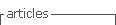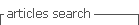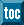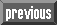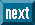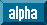## Indicators

•Cited by SciELO
•Access statistics

•Cited by Google
•Similars in SciELO
•Similars in Google

## Print version ISSN 0370-3908

#### Abstract

COSSIO, Jorge. Existence and multiplicity of solutions to semilinear and quasilinear elliptic problems. Rev. acad. colomb. cienc. exact. fis. nat. [online]. 2015, vol.39, n.151, pp.138-151. ISSN 0370-3908.  https://doi.org/10.18257/raccefyn.193.

In this paper I present some results of my research studying existence, multiplicity, and qualitative properties of solutions to semilinear elliptic problems of the type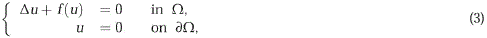and to quasilinear elliptic problems of the typewhere D is the Laplace operator, Dp is the p-Laplacian, W ⊂ RN (N ≥ 2) is a bounded domain in RN with smooth boundary, and f : R → R is a nonlinear function. Our theorems were obtained by using variational methods, a minmax principlie developed in Castro, Cossio & Neuberger (1997), Leray-Schauder degree, Morse theory, and bifurcation theory. Besides statement of principal results, we present some open problems which seem of interest for nonlinear analysts.

Keywords : Semilinear elliptic equations; quasilinear elliptic equations; variational methods; Lyapunov-Schmidt reduction; Leray-Schauder degree; Morse theory; bifurcation theory.

· abstract in Spanish     · text in Spanish     · Spanish (pdf )